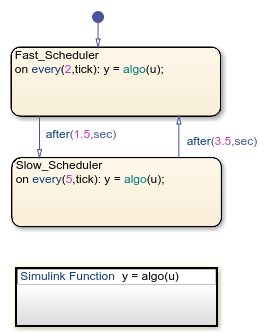Documentation

## Schedule Simulink Algorithms by Using Stateflow

This model shows how you can schedule a Simulink® algorithm using Stateflow®.The Stateflow chart in this model consists of a Simulink algorithm which runs at different rates at different times in the simulation. Stateflow is ideally suited to designing the transition logic which determines when these rate transitions happen. The Simulink algorithm on the other hand is used for the actual data processing. The chart merely passes inputs and outputs to the Simulink subsystem. For the purposes of this example, the Simulink algorithm adds the input to the output each time the chart calls it.Upon running the simulation, we see the counter counting at varying rates:## Related Topics

##### SupportGet trial now